# Prestress-loading effect on the current–voltage characteristics of a piezoelectric p–n junction together with the corresponding mechanical tuning laws

Department of Mechanics, Hubei Key Laboratory of Engineering Structural Analysis and Safety Assessment, Huazhong University of Science and Technology, Wuhan 430074, China

1. Corresponding author email

Associate Editor: E. Meyer
Beilstein J. Nanotechnol. 2019, 10, 1833–1843. https://doi.org/10.3762/bjnano.10.178
Received 21 May 2019, Accepted 23 Aug 2019, Published 06 Sep 2019

• Full Research Paper

## Abstract

Keywords: depletion layer; IV characteristics; piezoelectric property; p–n junction

## Introduction

In this paper, a model is proposed to study diffusion of NEMC in a mechanically loaded piezoelectric p–n junction with applied bias voltage. In section “IV characteristics of a mechanically loaded piezoelectric p–n junction”, the solving process for a low-level injection is divided into two steps: one is a quasi-electrostatic analysis of a mechanically loaded piezoelectric p–n junction under a bias voltage; and the other is to solve the continuity equation of NEMC by perturbation of small fields superposed on the obtained quasi-electrostatic solutions . Distributions of electric potential, electric field and carrier concentration are quasi-electrostatically solved in section “Quasi-electrostatic analysis of a mechanically loaded piezoelectric p–n junction under a bias voltage” for a piezoelectric p–n junction exposed to mechanical loading and electric bias voltages. IV characteristics are determined for a piezoelectric p–n junction under different mechanical loadings and with different bias voltages applied in section “Non-equilibrium analysis of a piezoelectric p–n junction under a bias voltage”. Numerical results show that a tuning can be achieved by mechanical loading. Finally, some general observations are summarized in section “Conclusion”.

## I–V characteristics of a mechanically loaded piezoelectric p–n junction

In conventional semiconductor physics , the IV characteristic of a common p–n junction under low-level injection is usually analyzed using a simple analogy based on the electrostatic analysis in thermal equilibrium, where the applied voltage (V) is assumed to drop in the depletion region such that its contact potential difference becomeswithbeing the initial voltage. It implies that the electric potential remains almost unchanged in the two zones outside the depletion layer, the p-zone and the n-zone, and the electric field is almost vanishing there. Thus, the steady-state diffusion equation of minority carriers in the two zones has no terms related to the electric field. However, the situation becomes different for a piezoelectric p–n junction under mechanical loading. The deformation-induced electric field together with its derivative appears in the continuity equation of minority carriers and influences the redistribution of minority carriers in each zone outside the SCZ. In turn, the redistribution of minority carriers influences the electric field itself. Under low-level electric injection, the effect of minority carriers on the electric field is much smaller than the influence of deformation. Hence, the IV characteristic of a mechanically loaded piezoelectric p–n junction under low-level injection can be obtained in through two steps (Figure 1). (I) Electric potential and electric field in both the p-zone and the n-zone induced by static loadings can be solved from the electrostatic analysis in thermal equilibrium; (II) the steady diffusion of minority carriers can be studied by using a perturbation technique of small fields superposed on the deformation-induced electric potential and electric field obtained in the first step.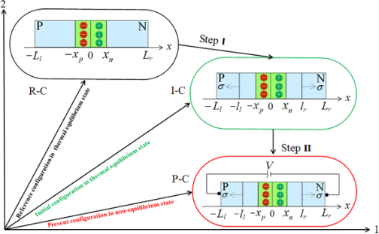Figure 1: Three configurations of a piezoelectric p–n junction: 1) R-C stands for the piezoelectric p–n junction in the reference configuration without mechanical loadings and any electric bias voltage; 2) I-C stands for the p–n junction in the initial configuration exposed to mechanical loading without any electric bias voltage; 3) P-C stands for the p–n junction in the present configuration with mechanical loading and an electric bias voltage V. Figure 1: Three configurations of a piezoelectric p–n junction: 1) R-C stands for the piezoelectric p–n junct... Jump to Figure 1

In step I, we solve the distributions of electric potential field, electric field and carrier concentrations for a piezoelectric p–n junction in the I-C state shown in Figure 1. According to  the location of the loading has a great influence. In order to mechanically tune the performance of a piezoelectric p–n junction, the prestress should need to be applied close to the SCZ. A piezoelectric p–n junction can be divided into six parts schematically shown in the I-C state in Figure 1, where the c-axis of both p-zone and n-zone is set in the x-direction. Tensile/compressive stress, σ, is applied at x = −ll and x = lr. The coordinates −xp and xn are two boundaries of the SCZ. For −ll < x < lr, the electric displacement can be written aswhere σ = cSeE,and ϑ2 = e2/(cε). In the above, strain S and electric field E can be expressed as S = du/dx and E = −dϕ/dx, in which u and ϕ are displacement and electric potential, respectively. c, e and ε are elastic, piezoelectric and dielectric constant, respectively.

The one-dimensional Gauss’s law of a piezoelectric p–n junction can be written as

where (p(n0,p0)(x), n(n0,p0)(x)) and (NA, ND) are carrier concentrations and doping concentrations, respectively. The subscripts “p” and “n” represent p-zone and n-zone, respectively. q = 1.602 × 10−19 C is the electronic charge. For a piezoelectric p–n junction exposed to stress at x = −ll and x = lr, the carrier concentrations can be obtained from the vanishing current requirements in thermal equilibrium 

in which k is the Boltzmann constant, T represents temperature (as 300 K in our analysis) and(pp0, np0) and (pn0, nn0) are initial hole and electron concentrations in the p-zone and n-zone, respectively. ni stands for the intrinsic carrier concentration in the thermal equilibrium state. Based on the depletion layer hypothesis, we obtain the charge balance condition

In addition, the contact potential difference of SCZ under nonzero σ can be solved as

It should be noted that D = 0 on x = –Ll and x = Lr has been applied in the above.

In step II, an electric bias voltage V is applied on the p–n junction shown in the P-C state in Figure 1. Under the condition of low-level injection, the applied electric bias voltage is assumed to drop completely in the SCZ in conventional semiconductor physics . Considering that the change of the electric field caused by NEMC under the low-level injection is very small, we still assume the applied electric bias voltage to vanish completely in the SCZ. Obviously, distributions of majority carriers and electric field are scarcely influenced by diffusion of minority carriers. The electric potential field ϕ and electric field –dϕ/dx are solved similarly as in step I just by replacingwithThus, the current density from diffusion of minority carriers in p-zone and n-zone can be expressed as

In the above,denotes NEMC in the p-zone anddenotes NEMC in the n-zone; jn and jp are the minority current densities, respectively. (µp, Dp) and (µn, Dn) are mobility and diffusion coefficients of holes and electrons, respectively.

The continuity equation of NEMC can be obtained as follows by perturbation of small fields superposed upon step I 

where (τn, τp) are the recombination lifetimes of electrons and holes, respectively. A forward-bias voltage lowers the potential barrier of the p–n junction such that majority carriers in the n- and p-zone, go across the SCZ to accumulate on the boundary at the other side as NEMC. The accumulation of minority carriers there will give rise to gradients of NEMC, which stimulates diffusion of NEMC departing from the two boundaries into the zones outside the SCZ and causes a positive current; a reverse-bias voltage increases the potential barrier such that minority carriers at the boundaries are extracted across the SCZ to form a reversal current. We note that the current across a piezoelectric p–n junction can be solved from Equation 6 together with the following boundary conditions : 1) no NEMC at x = –Ll and x = Lr; 2) continuity of NEMC and current density at x = –ll and x = lr; 3) NEMC concentrations at the SCZ boundaries satisfy

## Results and Discussion

### Quasi-electrostatic analysis of a mechanically loaded piezoelectric p–n junction under a bias voltage

Firstly, electric potential and electric field in a mechanically loaded piezoelectric p–n junction under a bias voltage are studied from a quasi-electrostatic analysis similar to step I. The bias voltage is assumed to act on the SCZ under the condition of low-level injection. The material constants of CdS rods with c-axis in x-direction are from [34,35]: c = 93.8 GPa, e = 0.44 C/m2, ε = 9.53ε0 and ε0 = 8.8542 × 10−12 F/m. The relative carrier concentrations in our calculations are taken as pp0 = nn0 = 1 × 1021 m−3 and np0 = pn0 = 1 × 1011 (m−3), unless otherwise stated. The doping concentrations can be expressed as NA = ND = 1 × 1021 m−3. The lengths involved are taken as Ll = Lr = L = 10ll,unless otherwise stated.andare the initial SCZ boundaries without mechanical loading or electric bias voltage. Due to the same doping,is obtained from Equation 3. They can be determined from  as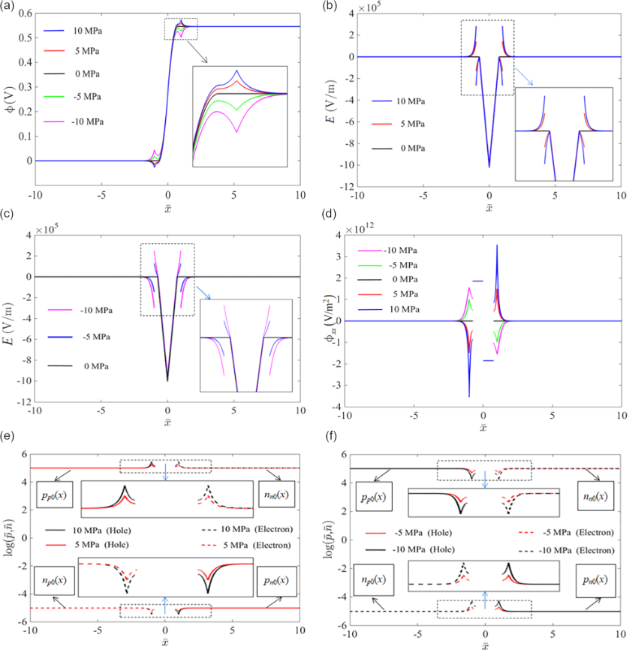Figure 2: Quasi-electrostatic analysis of a mechanically loaded piezoelectric p–n junction under a bias voltage: (a) electric potential; (b) electric field under tensile stress; (c) electric field under compressive stress; (d) the second derivation of electric potential; (e) carrier concentrations under tensile stress; (f) carrier concentrations under compressive stress. Figure 2: Quasi-electrostatic analysis of a mechanically loaded piezoelectric p–n junction under a bias volta... Jump to Figure 2

### Non-equilibrium analysis of a piezoelectric p–n junction under a bias voltage

The mobility coefficients of holes and electrons of CdS at 300 K are (µp, µn) = (0.005, 0.034) m2/V·s [34,35], and the corresponding diffusion constants can be obtained from the Einstein relation as (Dp, Dn) = (µp, µn)kT/q. In the following, we will focus on the influence of different mechanical loadings, different loading locations and different doping concentrations on the IV characteristics of a piezoelectric p–n junction .

#### Effect of mechanical loading on I–V characteristics of a piezoelectric p–n junction

In this section, we discuss the effect of mechanical loading on the IV characteristics of a piezoelectric p–n junction under forward- or reverse-bias voltages. Regarding the recombination lifetime of minority carriers, we take τn = τp = τ = 1 × 10−9 s to analyze the IV characteristics of a CdS p–n junction with NA = ND = 1 × 1021 m−3. To examine the validity of our analysis model, we first calculate the IV characteristics of the p–n junction without mechanical loading in Figure 3. The results based on the Shockley model for a pure semiconductor p–n junction is also given in the plot for comparison. Our results agree with those obtained from the Shockley model under both forward- and reversal-bias voltages. In the following, we will therefore conduct computations on the IV characteristics using our model.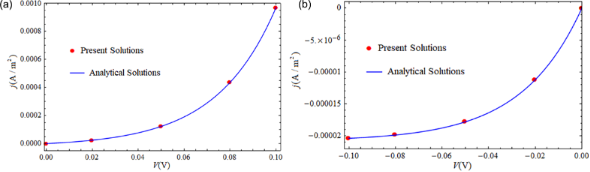Figure 3: Comparison of I–V characteristics obtained from our model and the Shockley model under (a) forward-bias voltages; (b) reverse-bias voltages. Figure 3: Comparison of I–V characteristics obtained from our model and the Shockley model under (a) forward-... Jump to Figure 3

Figure 5 further illustrates the phenomenon of reverse current density caused by tensile loading under small forward-bias voltages. As shown in Figure 5a, the negative current density appears at a tensile loading of 3.6 MPa for a 0.01 V forward-bias voltage and at a tensile loading of 8.6 MPa for a 0.02 V forward-bias voltage. The distributions of NEMC for different tensile loadings are shown in Figure 5b with a forward-bias voltage V = 0.02 V. Obviously, the concentrations of minority carriers at the SCZ boundaries under a forward-bias voltage of V = 0.02 V for σ < 8.6 MPa are larger than the ones in thermal equilibrium, i.e.,andwhich implies the appearance of an injection mode of NEMC. Concentrations of minority carriers at the SCZ boundaries under a forward-bias voltage of V = 0.02 V for σ > 8.6 MPa are smaller than the ones in thermal equilibrium, i.e.,andwhich implies appearance of an extraction mode of NEMC.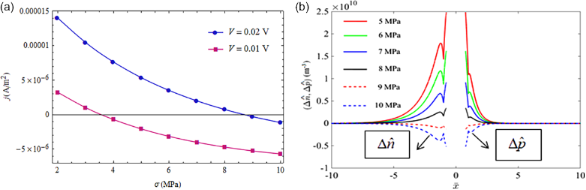Figure 5: (a) Current density as a function of applied tensile stress under two constant forward-bias voltages; (b) NEMC under different tensile stresses at V = 0.02 V. Figure 5: (a) Current density as a function of applied tensile stress under two constant forward-bias voltage... Jump to Figure 5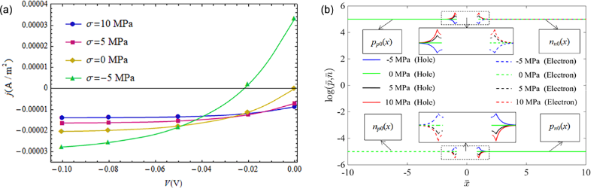Figure 6: (a) I–V characteristics of a piezoelectric p–n junction under reverse-bias voltages and different applied stresses; (b) distribution of the initial carrier concentrations for V = −0.10 V. Figure 6: (a) I–V characteristics of a piezoelectric p–n junction under reverse-bias voltages and different a... Jump to Figure 6

Figure 7a shows that the current density reverses from negative to positive under increasing compressive loading and a constant reverse-bias voltage. As mentioned above, a compressive loading is equivalent to a forward-bias voltage due to the piezoelectric properties of CdS. This equivalent forward-bias voltage will counteract on the reverse-bias voltage. Once the compressive stress increases to a certain value the equivalent forward-bias voltage is larger than the applied reverse-bias and the direction reversal happens for the current density of the p–n junction, for example at σ = −2.7 MPa and V = −0.01 V and at σ = −4.7 MPa and V = −0.02 V. The corresponding distribution of NEMC is shown in Figure 7b for V = −0.02 V. It can be seen that change of NEMC at the SCZ boundaries changes from extraction mode to injection mode when the applied compressive stress increases over −5 MPa. This direction reversal of the current density may also be of significance in force sensors.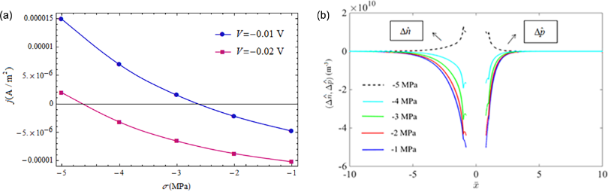Figure 7: Positive current density under a reverse-bias voltage. (a) Current density under different applied compressive stresses and a fixed reverse-bias voltage; (b) NEMC under different compressive stresses at V = −0.02 V. Figure 7: Positive current density under a reverse-bias voltage. (a) Current density under different applied ... Jump to Figure 7

#### Influence of loading locations on the I–V characteristics of a CdS p–n junction under forward-bias voltages

As reported in , the distance between the loading points and the SCZ boundaries exhibit great influence on the electrostatic quantities, that is, loading with a shorter distance produces a stronger influence on distributions of electric potential and carrier concentrations near the SCZ. Figure 8a shows this influence for compressive loading, σ = −10 MPa, and Figure 8b for tensile loading, σ = 10 MPa. It can be seen from Figure 8 that all IV characteristics approach that without mechanical loading (σ = 0 MPa) when l increases fromtoThe polarization electric field is disturbed once the piezoelectric semiconductors are exposed to prestress/prestrain. Thus, the carriers will be redistributed to induce an additional electric field, which in turn has a weakening effect on the polarization electric field. Obviously, the above two electric fields will offset each other in a sufficiently large action region, i.e., at longer distances between loading point and SCZ boundary.

#### The I–V characteristics of a CdS p–n junction with different doping concentrations under forward-bias voltages

In the above analysis, doping concentrations of a CdS p–n junction were kept constant at NA = ND = 1 × 1021 m−3. In this section, we study effect of the doping concentration on the IV characteristics of a CdS p–n junction by considering another doping concentration, i.e., NA = ND = 1 × 1022 m−3. The lifetime of non-equilibrium carriers reduces with increasing doping concentrations . Thus, the corresponding recombination lifetime is taken as τn = τp = τ = 1 × 10−10 s for NA = ND = 1 × 1022 m−3. The loading location is set at l =in Figure 9a–c and set at l =in Figure 9d, whereis calculated similar as above just by replacing the doping concentrations and the contact potential difference with those corresponding to NA = ND = 1 × 1022 (m−3).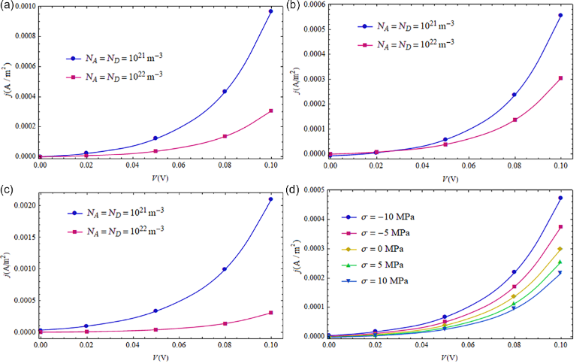Figure 9: I–V characteristics of a CdS p–n junction with different doping concentrations exposed to mechanical stress: (a) σ = 0 MPa. (b) σ = 5 MPa. (c) σ = −5 MPa. (d) Effect of mechanical loadings on a CdS p–n junction with NA = ND = 1 × 1022 m−3. Figure 9: I–V characteristics of a CdS p–n junction with different doping concentrations exposed to mechanica... Jump to Figure 9

The IV characteristics of a CdS p–n junction with different doping concentrations and mechanical loadings are shown in Figure 9. Obviously, the larger doping concentrations correspond to the higher potential barrier of the SCZ. Thus, a greater current density is obtained from the lower doping concentrations (Figure 9a). We note from  that the semiconducting property of a piezoelectric medium leads to electric leakage, which weakens the deformation-induced electric field. Thus, the higher doping concentration yields a lower current density for a given bias voltage, as shown in Figure 9b and 9c. Figure 9d shows the mechanical tuning for a CdS p–n junction with NA = ND = 1 × 1022 m−3. A compressive loading increases the output current density while a tensile loading decreases the output current density. This phenomenon can be used to design newly piezotronic devices.

## Acknowledgements

This work was supported by the National Natural Science Foundation of China (Grant Nos. 11672113, 11972164 and 51435006) and the Key Laboratory Project of Hubei Province (No. 2016CFA073).

#### Interesting articles

##### Piezotronic effect in AlGaN/AlN/GaN heterojunction nanowires used as a flexible strain sensor

Jianqi Dong, Liang Chen, Yuqing Yang and Xingfu Wang

##### Cr(VI) remediation from aqueous environment through modified-TiO2-mediated photocatalytic reduction

Rashmi Acharya, Brundabana Naik and Kulamani Parida

##### Graphene composites with dental and biomedical applicability

Sharali Malik, Felicite M. Ruddock, Adam H. Dowling, Kevin Byrne, Wolfgang Schmitt, Ivan Khalakhan, Yoshihiro Nemoto, Hongxuan Guo, Lok Kumar Shrestha, Katsuhiko Ariga and Jonathan P. Hill

#### News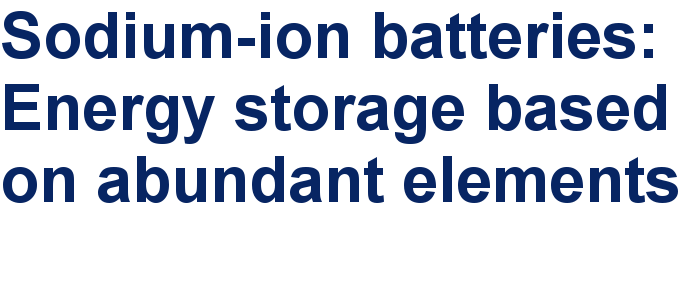##### Take a look at the top five most cited articles on the topic perovskites and perovskite solar cells.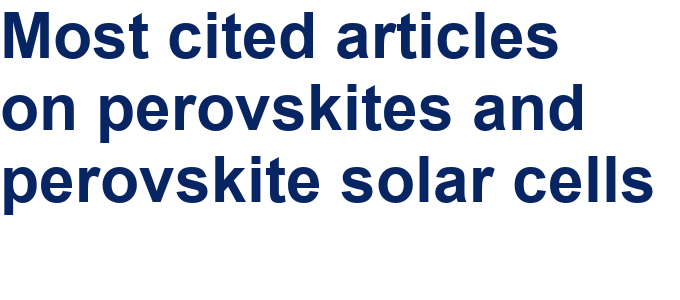##### Professor Xiaoyan Song joins the Editorial Board of the Beilstein Journal of NanotechnologyThis is an Open Access article under the terms of the Creative Commons Attribution License (http://creativecommons.org/licenses/by/4.0). Please note that the reuse, redistribution and reproduction in particular requires that the authors and source are credited.
The license is subject to the Beilstein Journal of Nanotechnology terms and conditions: (https://www.beilstein-journals.org/bjnano)